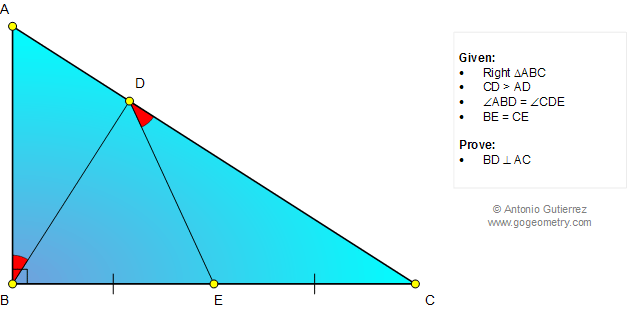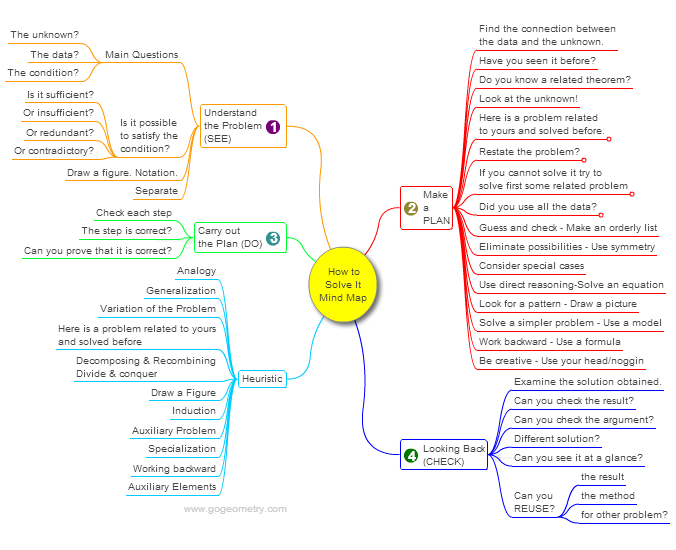# Online Math: Geometry Problem 1029: Right Triangle, 90 Degrees, Angle, Midpoint, Congruence, Perpendicular. Level: School, College.

< PREVIOUS PROBLEM  |  NEXT PROBLEM >

The figure below shows a right triangle ABC with D on AC and E on BC so that BE = CE, CD greater than AD, and angle ABD = angle CDE. Prove that BD is perpendicular to AC.## Mind Map: George Pólya's 1945 book, "How to Solve It"

George Pólya's 1945 book "How to Solve It, A new aspect of Mathematical Method", is a book describing methods of problem solving. It suggests the following steps when solving a mathematical problem: (1) First, you have to understand the problem. (2) After understanding, then make a plan. (3) Carry out the plan. (4) Look back on your work. How could it be better?
A mind map is a diagram used to visually organize information, knowledge, concepts or ideas.Home | SearchGeometry | Problems | All Problems | Open Problems | Visual Index | 10 Problems | Problems Art Gallery Art | 1021-1030 | Triangle | Midpoint | Congruence | Perpendicular lines | Email

Add a solution to the problem 1029
Last updated: Sep 19, 2014